### Example 84.1 Testing the Difference between Two Proportions

This example demonstrates group sequential tests that use an O’Brien-Fleming group sequential design. A clinic is studying the effect of vitamin C supplements in treating flu symptoms. The study consists of patients in the clinic who have exhibited the first sign of flu symptoms within the last 24 hours. These patients are randomly assigned to either the control group (which receives placebo pills) or the treatment group (which receives large doses of vitamin C supplements). At the end of a five-day period, the flu symptoms of each patient are recorded.

Suppose that you know from past experience that flu symptoms disappear in five days for 60% of patients who experience flu symptoms. The clinic would like to detect a 75% symptom disappearance with a high probability. A test that compares the proportions directly specifies the null hypothesis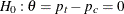with a one-sided alternative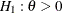and a power of 0.90 at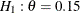, where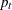and
are the proportions of symptom disappearance in the treatment group and control group, respectively.

The following statements invoke the SEQDESIGN procedure and request a four-stage group sequential design by using an O’Brien-Fleming method for normally distributed data. The design uses a one-sided alternative hypothesis with early stopping either to accept or reject the null hypothesis. The BOUNDARYSCALE=MLE option uses the MLE scale to display statistics in the boundary table and boundary plots.

ods graphics on;
proc seqdesign altref=0.15
boundaryscale=mle
;
OBrienFleming: design method=obf
nstages=4
alt=upper
stop=both
alpha=0.025
;
samplesize model=twosamplefreq(nullprop=0.6 test=prop);
ods output Boundary=Bnd_Count;
run;
ods graphics off;


The ODS OUTPUT statement with the BOUNDARY=BND_COUNT option creates an output data set named BND_COUNT which contains the resulting boundary information for the subsequent sequential tests.

The Design Information table in Output 84.1.1 displays design specifications. With the specified alternative hypothesis, the maximum information is derived to achieve a power of 0.90 at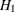. The derived fixed-sample information ratio 1.0767 is the maximum information needed for a group sequential design relative to its corresponding fixed-sample design.

The SEQDESIGN Procedure
Design: OBrienFleming

Statistic Distribution Normal
Boundary Scale MLE
Alternative Hypothesis Upper
Early Stop Accept/Reject Null
Method O'Brien-Fleming
Boundary Key Both
Alternative Reference 0.15
Number of Stages 4
Alpha 0.025
Beta 0.1
Power 0.9
Max Information (Percent of Fixed Sample) 107.6741
Max Information 502.8343
Null Ref ASN (Percent of Fixed Sample) 61.12891
Alt Ref ASN (Percent of Fixed Sample) 75.89782

The Boundary Information table in Output 84.1.2 displays the information level, alternative reference, and boundary values at each stage. With the BOUNDARYSCALE=MLE option, the SEQDESIGN procedure displays the output boundaries with the maximum likelihood estimator scale.

Output 84.1.2: O’Brien-Fleming Boundary Information

Boundary Information (MLE Scale)
Null Reference = 0
_Stage_   Alternative Boundary Values
Information Level Reference Upper
Proportion Actual N Upper Beta Alpha
1 0.2500 125.7086 107.4808 0.15000 -0.09709 0.35291
2 0.5000 251.4171 214.9617 0.15000 0.02645 0.17645
3 0.7500 377.1257 322.4425 0.15000 0.06764 0.11764
4 1.0000 502.8343 429.9233 0.15000 0.08823 0.08823

With ODS Graphics enabled, a detailed boundary plot with the rejection and acceptance regions is displayed, as shown in Output 84.1.3. The horizontal axis indicates the information levels for the design. The stages are indicated by vertical lines with accompanying stage numbers. If the test statistic at a stage is in a rejection region, the trial stops and the hypothesis is rejected. If the test statistic is in an acceptance region, then the trial also stops and the hypothesis is accepted. If the statistic is not in a rejection or an acceptance region, the trial continues to the next stage.

Output 84.1.3: O’Brien-Fleming Boundary Plot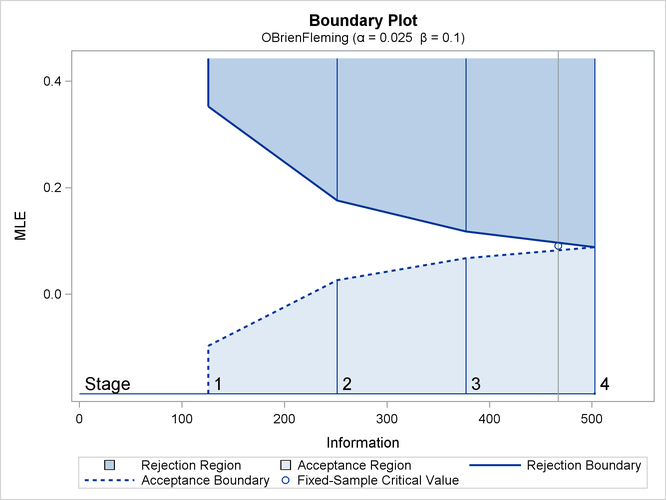The boundary plot also displays the information level and critical value for the corresponding fixed-sample design. The solid and dashed lines at the fixed-sample information level correspond to the rejection and acceptance lines, respectively.

With the SAMPLESIZE statement, the maximum information is used to derive the required sample size for the study. The Sample Size Summary table in Output 84.1.4 displays parameters for the sample size computation.

Output 84.1.4: Required Sample Size Summary

Sample Size Summary
Test Two-Sample Proportions
Null Proportion 0.6
Proportion (Group A) 0.75
Test Statistic Z for Proportion
Reference Proportions Alt Ref
Max Sample Size 429.9233
Expected Sample Size (Null Ref) 244.0768
Expected Sample Size (Alt Ref) 303.0464

With the derived maximum information and the specified MODEL= option in the SAMPLESIZE statement, the total sample size in each group for testing the difference between two proportions under the alternative hypothesis is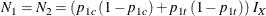where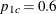and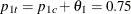. By default (or equivalently if you specify REF=PROP in the MODEL=TWOSAMPLEFREQ option), the required sample sizes are computed under the alternative hypothesis. See the section Test for the Difference between Two Binomial Proportions in the chapter The SEQDESIGN Procedure for a description of these parameters.

The Sample Sizes (N) table in Output 84.1.5 displays the required sample sizes at each stage, in both fractional and integer numbers. The derived sample sizes under the heading Fractional N which correspond to the design are not integers. These sample sizes are rounded up to integers under the heading Ceiling N. In practice, integer sample sizes are used, and the information levels increase slightly. Thus, 54, 108, 162, and 215 patients are needed in each group for the four stages, respectively.

Output 84.1.5: Required Sample Sizes

Sample Sizes (N)
Two-Sample Z Test for Proportion Difference
_Stage_ Fractional N Ceiling N
N N(Grp 1) N(Grp 2) Information N N(Grp 1) N(Grp 2) Information
1 107.48 53.74 53.74 125.7 108 54 54 126.3
2 214.96 107.48 107.48 251.4 216 108 108 252.6
3 322.44 161.22 161.22 377.1 324 162 162 378.9
4 429.92 214.96 214.96 502.8 430 215 215 502.9

Suppose the trial follows the study plan, and 54 patients are available in each group at stage 1. The data set count_1 contains these 108 patients. Output 84.1.6 lists the first 10 observations of the data set.

Output 84.1.6: Clinical Trial Data

 First 10 Obs in the Trial Data

Obs Trt Resp
1 0 0
2 1 1
3 0 1
4 1 0
5 0 0
6 1 0
7 0 0
8 1 1
9 0 1
10 1 0

The Trt variable is a grouping variable with value 0 for a patient in the placebo control group and value 1 for a patient in the treatment group who is given vitamin C supplements. The Resp variable is an indicator variable with value 1 for a patient without flu symptoms after five days and value 0 for a patient with flu symptoms after five days.

The following statements use the GENMOD procedure to estimate the treatment effect at stage 1:

proc genmod data=count_1;
model Resp= Trt;
ods output ParameterEstimates=Parms_Count1;
run;


Output 84.1.7 displays the treatment effect at stage 1.

Output 84.1.7: Stage 1 Treatment Difference

The GENMOD Procedure

Analysis Of Maximum Likelihood Parameter Estimates
Parameter DF Estimate Standard Error Wald 95% Confidence Limits Wald Chi-Square Pr > ChiSq
Intercept 1 0.6296 0.0627 0.5066 0.7526 100.68 <.0001
Trt 1 0.1111 0.0887 -0.0628 0.2850 1.57 0.2105
Scale 1 0.4611 0.0314 0.4035 0.5269

 Note: The scale parameter was estimated by maximum likelihood.

The test statistic is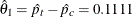, and its associated standard error is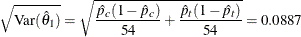The following statements create and display (in Output 84.1.8) the data set that contains the parameter estimate at stage 1,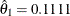, and its associated standard error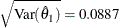which are used in the SEQTEST procedure:

data Parms_Count1;
set Parms_Count1;
if Parameter='Trt';
_Scale_='MLE';
_Stage_= 1;
keep _Scale_ _Stage_ Parameter Estimate StdErr;
run;

proc print data=Parms_Count1;
title 'Statistics Computed at Stage 1';
run;


Output 84.1.8: Statistics Computed at Stage 1

 Statistics Computed at Stage 1

Obs Parameter Estimate StdErr _Scale_ _Stage_
1 Trt 0.1111 0.0887 MLE 1

The initial required sample sizes are derived with the proportions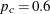and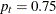. If the observed proportions are different from these assumed values, or if the number of available patients is different from the study plan in one of the stages, then the information level that corresponds to the test statistic is estimated from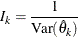The following statements invoke the SEQTEST procedure and test for early stopping at stage 1:

ods graphics on;
proc seqtest Boundary=Bnd_Count
Parms(Testvar=Trt)=Parms_Count1
errspendmin=0.001
boundaryscale=mle
errspend
plots=errspend
;
ods output Test=Test_Count1;
run;
ods graphics off;


The BOUNDARY= option specifies the input data set that provides the boundary information for the trial at stage 1, which was generated in the SEQDESIGN procedure. The PARMS=PARMS_COUNT1 option specifies the input data set PARMS_COUNT1 that contains the test statistic and its associated standard error at stage 1, and the TESTVAR=TRT option identifies the test variable TRT in the data set. The INFOADJ=NONE option maintains the information levels at future interim stages (2 and 3) as provided in the BOUNDARY= data set. The BOUNDARYSCALE=MLE option displays the output boundaries in terms of the MLE scale.

The O’Brien-Fleming design is conservative in early stages and might not be desirable in a clinical trial. The ERRSPENDMIN=0.001 option specifies the minimum error spending at each stage to be 0.001, and it might increase the corresponding nominal p-value in early stages for the trial. The BOUNDARYSCALE=MLE option uses the MLE scale to display test statistics in the boundary table and boundary plots.

The ODS OUTPUT statement with the TEST=TEST_COUNT1 option creates an output data set named TEST_COUNT1 which contains the updated boundary information for the test at stage 1. The data set also provides the boundary information that is needed for the group sequential test at the next stage.

The Design Information table in Output 84.1.9 displays design specifications. The derived statistics, such as the overallandlevels, are derived from the specified maximum information and boundary values in the BOUNDARY= data set. Note that with a minor change in the information level at stage 1, the power also changes slightly from the design provided in the BOUNDARY= data set.

The SEQTEST Procedure

BOUNDARY Data Set WORK.BND_COUNT
Data Set WORK.PARMS_COUNT1
Statistic Distribution Normal
Boundary Scale MLE
Alternative Hypothesis Upper
Early Stop Accept/Reject Null
Number of Stages 4
Alpha 0.025
Beta 0.10147
Power 0.89853
Max Information (Percent of Fixed Sample) 108.2301
Max Information 502.834283
Null Ref ASN (Percent of Fixed Sample) 61.09917
Alt Ref ASN (Percent of Fixed Sample) 73.9745

With the ERRSPEND option, the Error Spending Information table in Output 84.1.10 displays cumulative error spending at each stage for each boundary. By default (or equivalently if you specify BOUNDARYKEY=ALPHA), the Type I error level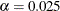is maintained. Furthermore, with the ERRSPENDMIN=0.001 option, thespending at each stage is greater than or equal to 0.001.

Output 84.1.10: Error Spending Information

Error Spending Information
_Stage_   Cumulative Error Spending
Information Level Upper
Proportion Actual Beta Alpha
1 0.2525 126.9871 0.00308 0.00100
2 0.5000 251.4171 0.02653 0.00343
3 0.7500 377.1257 0.06456 0.01254
4 1.0000 502.8343 0.10147 0.02500

With the PLOTS=ERRSPEND option, the procedure displays a plot of error spending for each boundary, as shown in Output 84.1.11. The error spending values in the Error Spending Information table in Output 84.1.10 are displayed in the plot.

Output 84.1.11: Error Spending Plot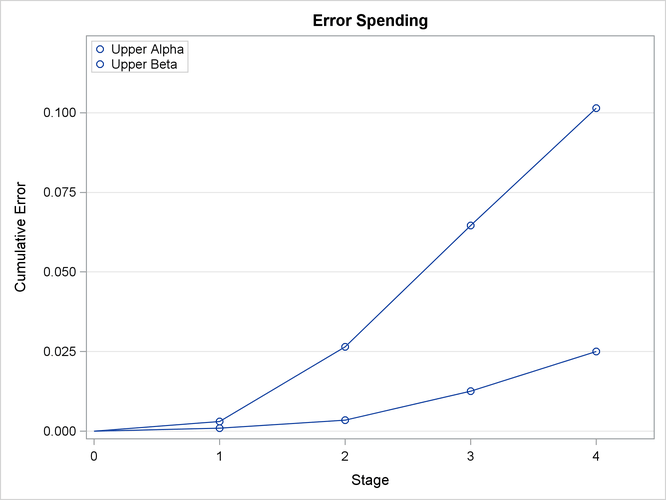The Test Information table in Output 84.1.12 displays the boundary values for the design, test statistic, and resulting action at each stage. With the BOUNDARYSCALE=MLE option, the maximum likelihood estimator scale is used for the test statistic and boundary values. The table shows that the test statistic 0.1111 is between the upperandboundaries, so the trial continues to the next stage.

Output 84.1.12: Sequential Test

Test Information (MLE Scale)
Null Reference = 0
_Stage_   Alternative Boundary Values Test
Information Level Reference Upper Trt
Proportion Actual Upper Beta Alpha Estimate Action
1 0.2525 126.9871 0.15000 -0.09306 0.27423 0.11111 Continue
2 0.5000 251.4171 0.15000 0.02674 0.17527 .
3 0.7500 377.1257 0.15000 0.06805 0.11792 .
4 1.0000 502.8343 0.15000 0.08875 0.08875 .

The information level at stage 1 is derived from the standard error,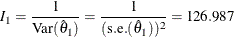By default (or equivalently if you specify PLOTS=TEST), the Test Plot graph displays boundary values of the design and the test statistic at stage 1, as shown in Output 84.1.13. It also shows that the observed statistic is in the continuation region.

Output 84.1.13: Sequential Test Plot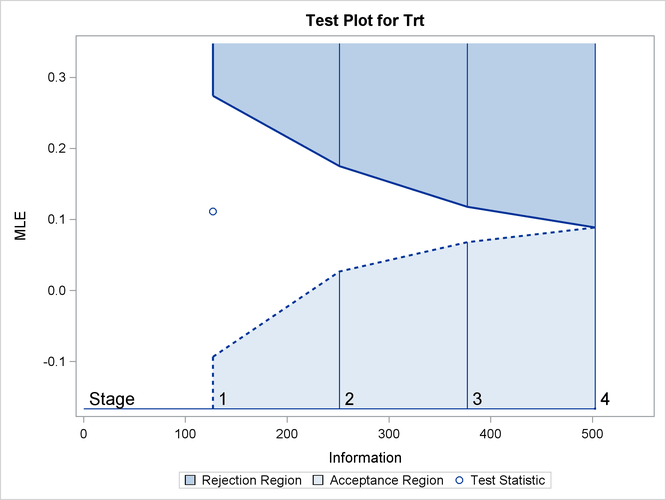The observed information level at stage 1,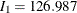, is slightly larger than the target information level at the design. If an observed information level in the study is substantially different from its target level in the design, then the sample sizes should be adjusted in the subsequent stages to achieve the target information levels.

Suppose the trial continues to the next stage, and 108 patients are available in each group at stage 2. The data set COUNT_2 contains these 216 patients.

The following statements use the GENMOD procedure to estimate the treatment effect at stage 2:

proc genmod data=Count_2;
model Resp= Trt;
ods output ParameterEstimates=Parms_Count2;
run;


The following statements create the parameter estimate at stage 2,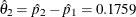, and its associated standard error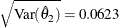into a test data set:

data Parms_Count2;
set Parms_Count2;
if Parameter='Trt';
_Scale_='MLE';
_Stage_= 2;
keep _Scale_ _Stage_ Parameter Estimate StdErr;
run;

proc print data=Parms_Count2;
title 'Statistics Computed at Stage 2';
run;


Output 84.1.14 displays the test statistics at stage 2.

Output 84.1.14: Statistics Computed at Stage 2

 Statistics Computed at Stage 2

Obs Parameter Estimate StdErr _Scale_ _Stage_
1 Trt 0.1759 0.0623 MLE 2

The following statements invoke the SEQTEST procedure and test for early stopping at stage 2:

ods graphics on;
proc seqtest Boundary=Test_Count1
Parms(Testvar=Trt)=Parms_Count2
boundaryscale=mle
;
ods output Test=Test_Count2;
run;
ods graphics off;


The BOUNDARY= option specifies the input data set that provides the boundary information for the trial at stage 2, which was generated by the SEQTEST procedure at the previous stage. The PARMS= option specifies the input data set that contains the test statistic and its associated standard error at stage 2, and the TESTVAR= option identifies the test variable in the data set.

The ODS OUTPUT statement with the TEST=TEST_COUNT2 option creates an output data set named TEST_COUNT2 which contains the updated boundary information for the test at stage 2. The data set also provides the boundary information that is needed for the group sequential test at the next stage.

The Test Information table in Output 84.1.15 displays the boundary values, test statistic, and resulting action at each stage. The table shows that the test statistic 0.17593 is larger than the corresponding upper alpha boundary value, so the trial stops to reject the hypothesis.

Output 84.1.15: Sequential Test

The SEQTEST Procedure

Test Information (MLE Scale)
Null Reference = 0
_Stage_   Alternative Boundary Values Test
Information Level Reference Upper Trt
Proportion Actual Upper Beta Alpha Estimate Action
1 0.2525 126.9871 0.15000 -0.09306 0.27423 0.11111 Continue
2 0.5122 257.5571 0.15000 0.03019 0.17001 0.17593 Reject Null
3 0.7500 377.1257 0.15000 0.06783 0.11826 .
4 1.0000 502.8343 0.15000 0.08878 0.08878 .

With ODS Graphics enabled, the Test Plot is displayed, as shown in Output 84.1.16. The plot displays boundary values of the design and the test statistics at the first two stages. As expected, the test statistic at stage 2 is in the Upper Rejection Region above the upper alpha boundary.

Output 84.1.16: Sequential Test Plot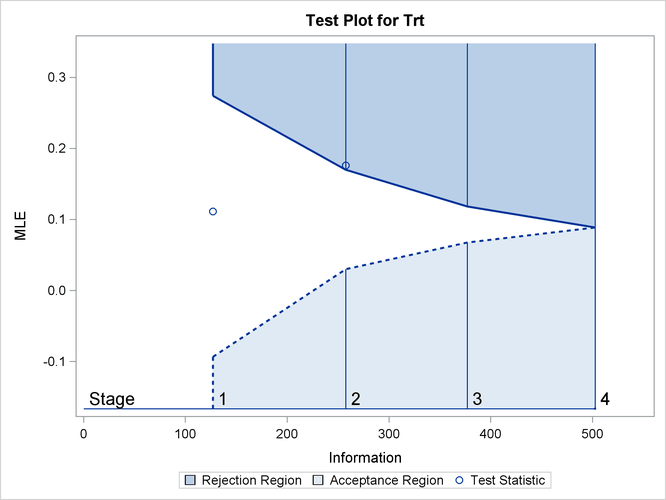After a trial is stopped, the Parameter Estimates table in Output 84.1.17 displays the stopping stage and the maximum likelihood estimate of the parameter. It also displays the p-value, median estimate, and confidence limits for the parameter that correspond to the observed statistic by using the specified sample space ordering.

Output 84.1.17: Parameter Estimates

Parameter Estimates
Stagewise Ordering
Parameter Stopping
Stage
MLE p-Value for
H0:Parm=0
Median
Estimate
Lower 95% CL
Trt 2 0.175926 0.0031 0.174462 0.07059

The MLE statistic at the stopping stage is the maximum likelihood estimate of the parameter and is biased. The computation of p-value, unbiased median estimate, and confidence limits depends on the ordering of the sample space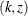, where k is the stage number and z is the observed standardized Z statistic. By default (or equivalently if you specify ORDER=STAGEWISE), the stagewise ordering that uses counterclockwise ordering around the continuation region is used to compute the p-value, unbiased median estimate, and confidence limits. As expected, the p-value is less than 0.025, and the confidence interval does not contain the null reference zero. With the stagewise ordering, the p-value is computed as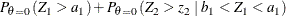where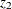is the observed standardized Z statistic at stage 2,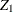is the standardized normal variate at stage 1,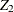is the standardized normal variate at stage 2, and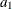and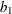are the stage 1 upper rejection and acceptance boundary values, respectively.

See the section Available Sample Space Orderings in a Sequential Test for a detailed description of the stagewise ordering.